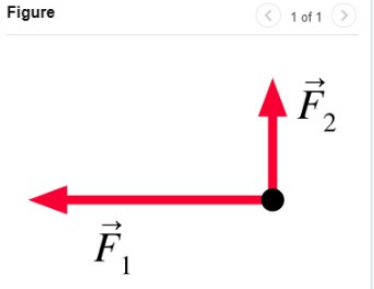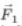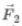# Problem: Two forces act on an object at rest as shown in (Figure 1)Add a third force that results in a net force of zero.Draw the vector starting at the black dot. The location, orientation, and length of the vector will be graded. You can move the vectors  and  to construct the required vector.

🤓 Based on our data, we think this question is relevant for Professor Dominguez's class at MDC.

###### FREE Expert Solution

The third force should be in a direction that opposes both F1 and F2.###### Problem Details

Two forces act on an object at rest as shown in (Figure 1)Add a third force that results in a net force of zero.

Draw the vector starting at the black dot. The location, orientation, and length of the vector will be graded. You can move the vectorsandto construct the required vector.